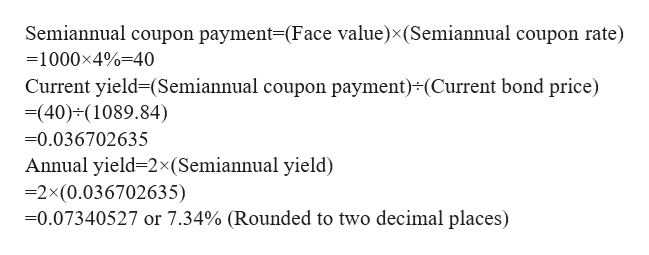# Brandt Corporation's 30-year bond has 10 years left until maturity. The 8% coupon bond has a par value of \$1000 and pays interest semiannually. The bond currently sells for \$1089.84.What is the bond's current yield?What is the bond's yield to maturity?

Question
8 views

Brandt Corporation's 30-year bond has 10 years left until maturity. The 8% coupon bond has a par value of \$1000 and pays interest semiannually. The bond currently sells for \$1089.84.

What is the bond's current yield?

What is the bond's yield to maturity?

check_circle

Step 1

Part 1:
We calculate the current yield by dividing the coupon payment by current price of the bond.

Step 2

Given that:
Face value is \$1000 and the current value of the bond is \$1089.84
Annual coupon rate is 8%, as the interest is paid semiannually, the semiannual coupon rate is 8% divided by 2 that is equal to 4%

Step 3help_outlineImage TranscriptioncloseSemiannual coupon payment-(Face value)x(Semiannual coupon rate) =1000x4%-40 Current yield-(Semiannual coupon payment) (Current bond price) (40) (1089.84) -0.036702635 Annual yield=2x(Semiannual yield) -2x(0.036702635) 0.07340527 or 7.34% (Rounded to two decimal places) fullscreen

### Want to see the full answer?

See Solution

#### Want to see this answer and more?

Solutions are written by subject experts who are available 24/7. Questions are typically answered within 1 hour.*

See Solution
*Response times may vary by subject and question.
Tagged in